# Perpindahan panas

2. Dec 2012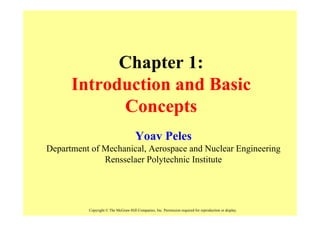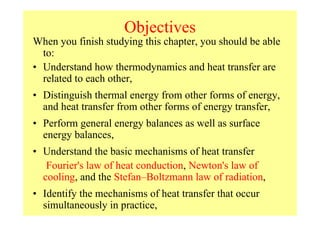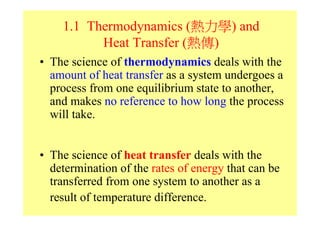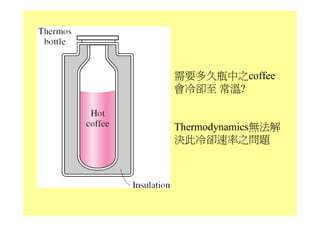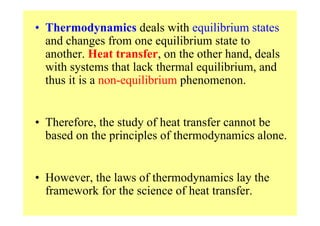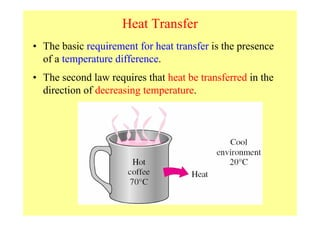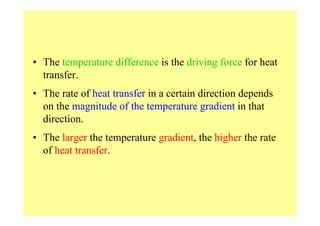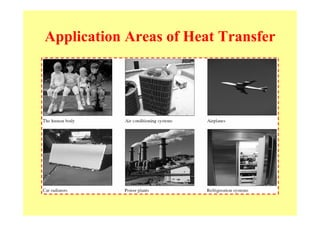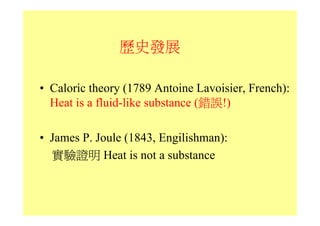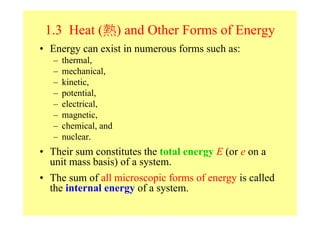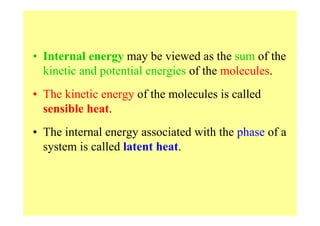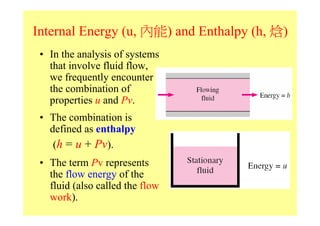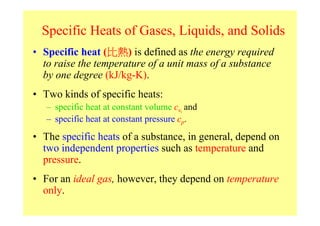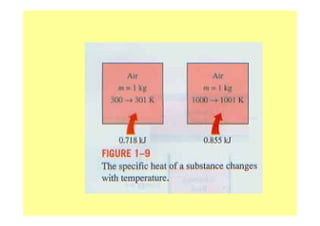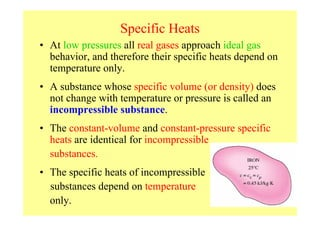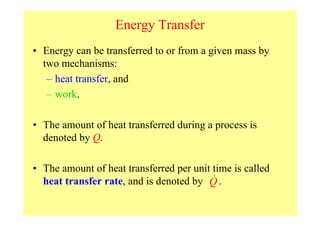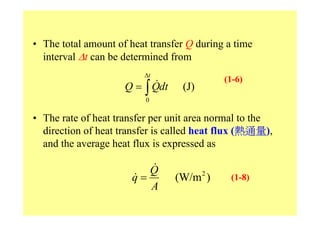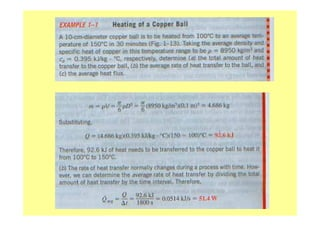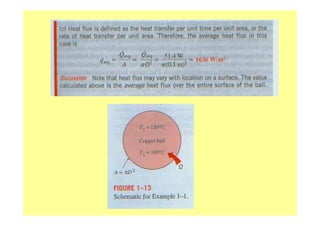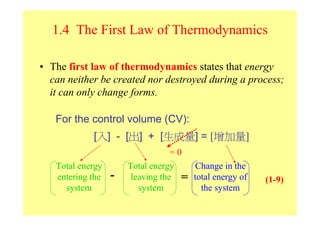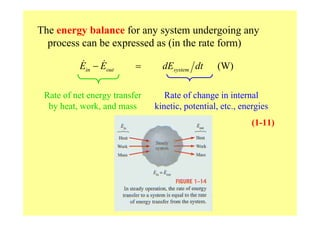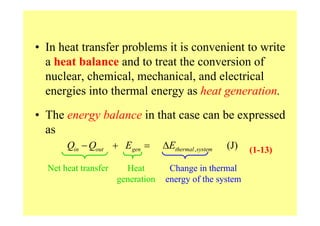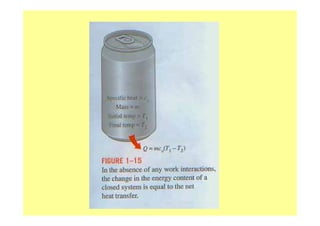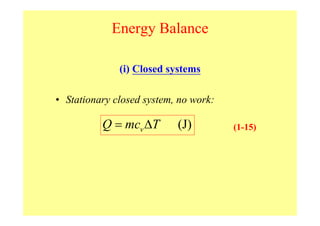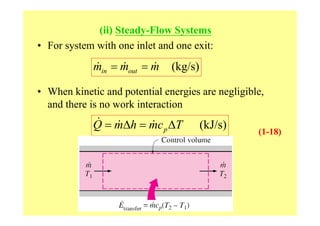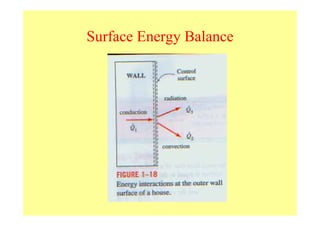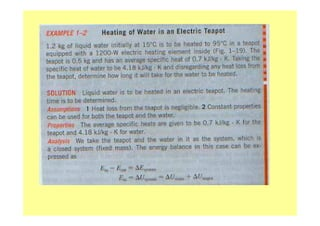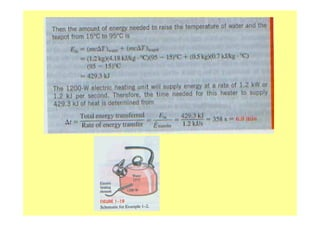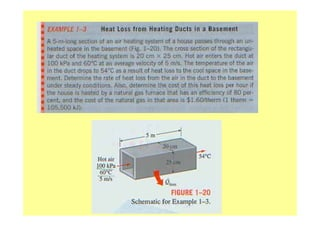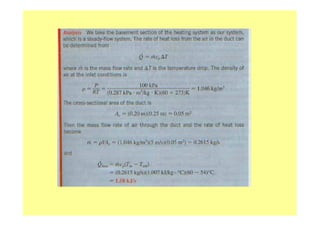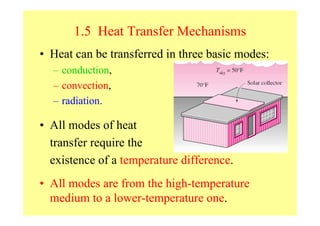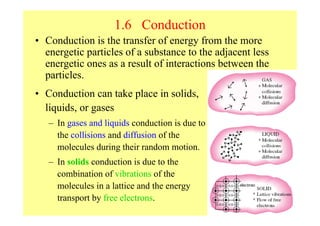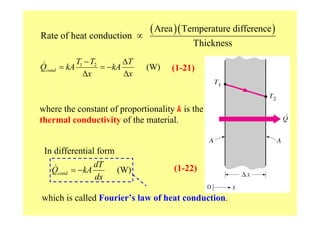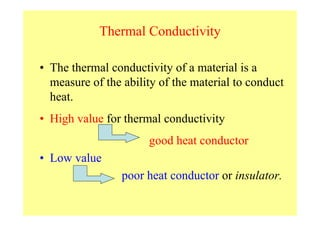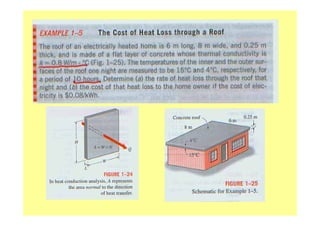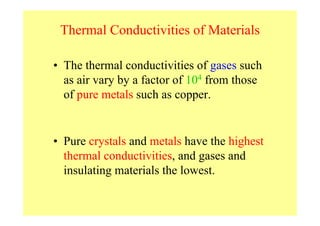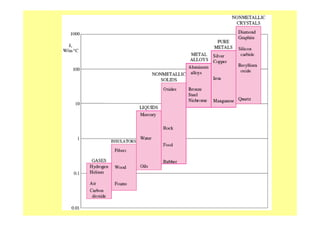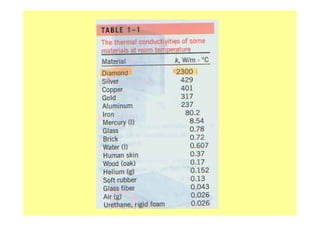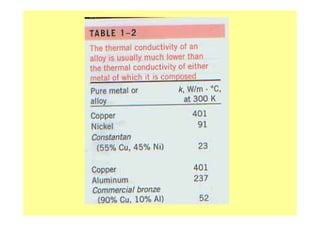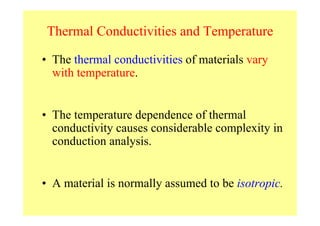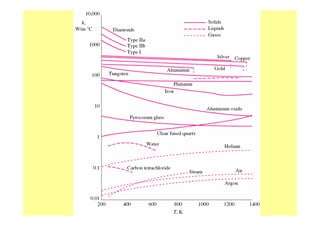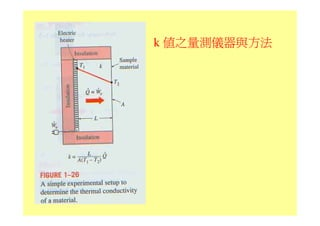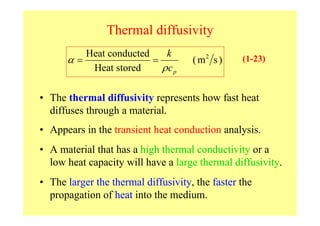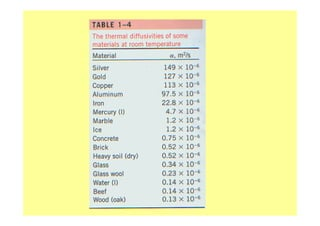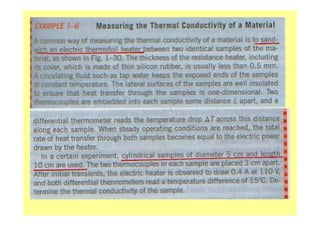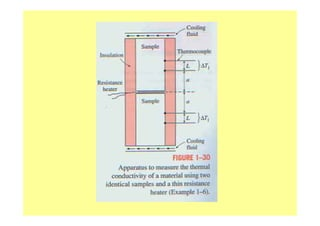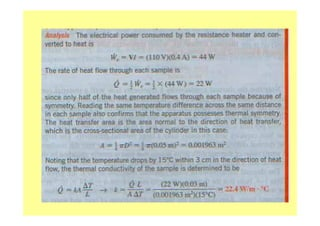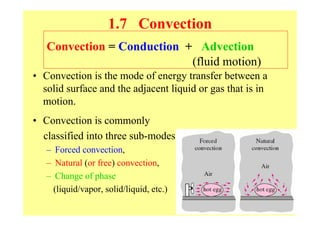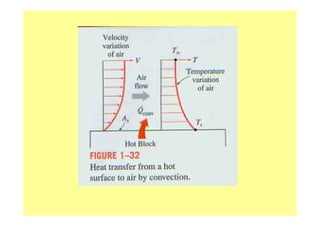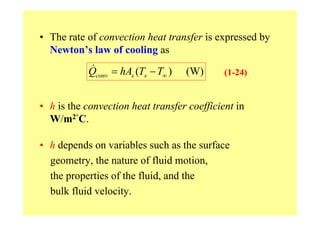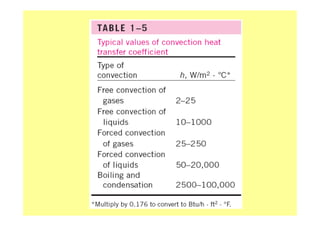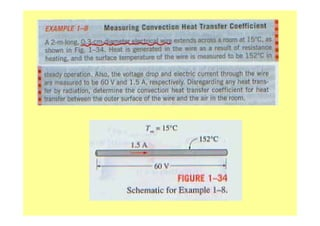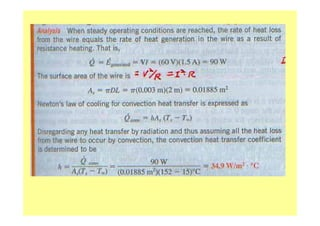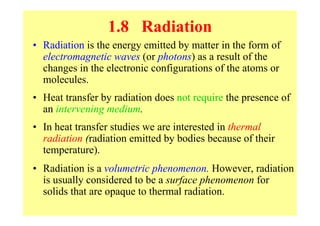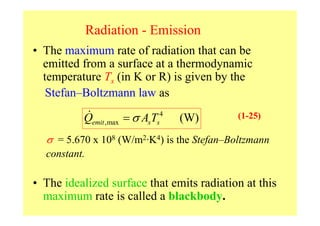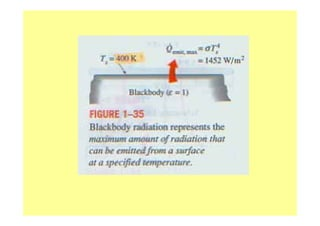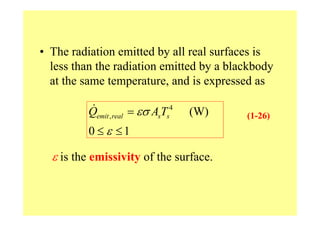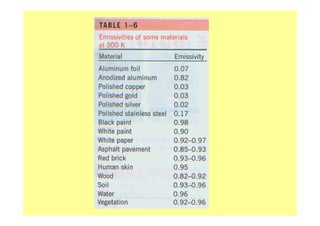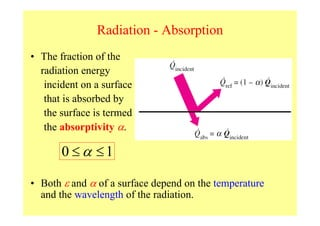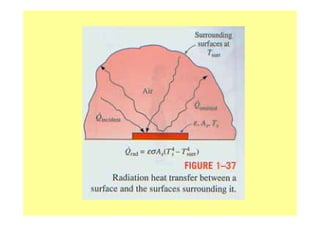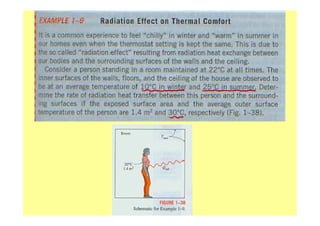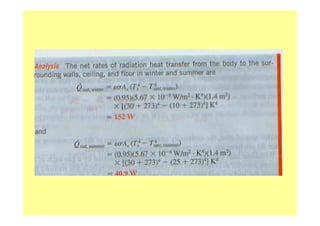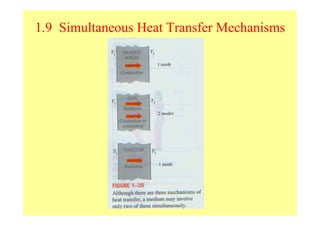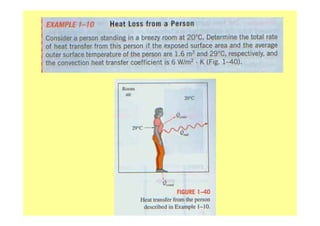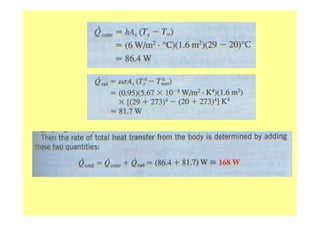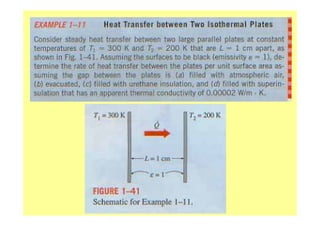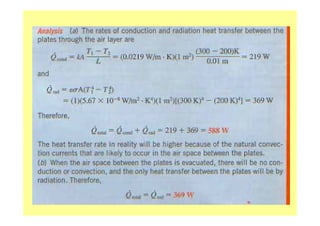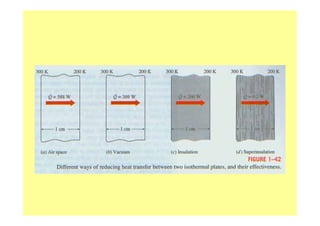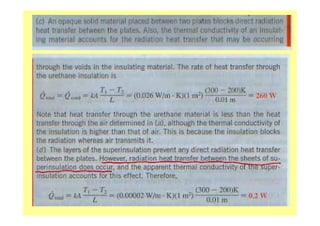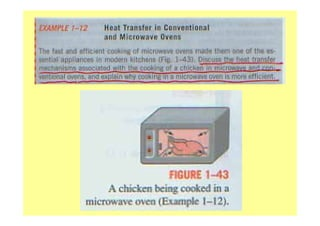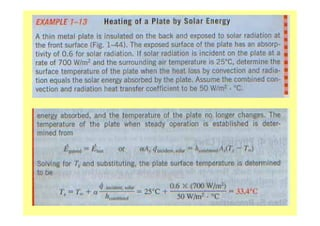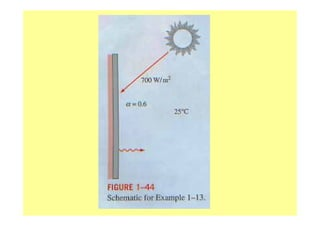1 von 74

### Perpindahan panas

• 1. Chapter 1: Introduction and Basic Concepts Yoav Peles Department of Mechanical, Aerospace and Nuclear Engineering Rensselaer Polytechnic Institute Copyright © The McGraw-Hill Companies, Inc. Permission required for reproduction or display.
• 2. Objectives When you finish studying this chapter, you should be able to: • Understand how thermodynamics and heat transfer are related to each other, • Distinguish thermal energy from other forms of energy, and heat transfer from other forms of energy transfer, • Perform general energy balances as well as surface energy balances, • Understand the basic mechanisms of heat transfer Fourier's law of heat conduction, Newton's law of cooling, and the Stefan–Boltzmann law of radiation, • Identify the mechanisms of heat transfer that occur simultaneously in practice,
• 3. 1.1 Thermodynamics (熱力學) and Heat Transfer (熱傳) • The science of thermodynamics deals with the amount of heat transfer as a system undergoes a process from one equilibrium state to another, and makes no reference to how long the process will take. • The science of heat transfer deals with the determination of the rates of energy that can be transferred from one system to another as a result of temperature difference.
• 5. • Thermodynamics deals with equilibrium states and changes from one equilibrium state to another. Heat transfer, on the other hand, deals with systems that lack thermal equilibrium, and thus it is a non-equilibrium phenomenon. • Therefore, the study of heat transfer cannot be based on the principles of thermodynamics alone. • However, the laws of thermodynamics lay the framework for the science of heat transfer.
• 6. Heat Transfer • The basic requirement for heat transfer is the presence of a temperature difference. • The second law requires that heat be transferred in the direction of decreasing temperature.
• 7. • The temperature difference is the driving force for heat transfer. • The rate of heat transfer in a certain direction depends on the magnitude of the temperature gradient in that direction. • The larger the temperature gradient, the higher the rate of heat transfer.
• 8. Application Areas of Heat Transfer
• 9. 歷史發展 • Caloric theory (1789 Antoine Lavoisier, French): Heat is a fluid-like substance (錯誤!) • James P. Joule (1843, Engilishman): 實驗證明 Heat is not a substance
• 10. 1.3 Heat (熱) and Other Forms of Energy • Energy can exist in numerous forms such as: – thermal, – mechanical, – kinetic, – potential, – electrical, – magnetic, – chemical, and – nuclear. • Their sum constitutes the total energy E (or e on a unit mass basis) of a system. • The sum of all microscopic forms of energy is called the internal energy of a system.
• 11. • Internal energy may be viewed as the sum of the kinetic and potential energies of the molecules. • The kinetic energy of the molecules is called sensible heat. • The internal energy associated with the phase of a system is called latent heat.
• 12. • The internal energy associated with the atomic bonds in a molecule is called chemical (or bond) energy. • The internal energy associated with the bonds within the nucleus of the atom itself is called nuclear energy.
• 13. Internal Energy (u, 內能) and Enthalpy (h, 焓) • In the analysis of systems that involve fluid flow, we frequently encounter the combination of properties u and Pv. • The combination is defined as enthalpy (h = u + Pv). • The term Pv represents the flow energy of the fluid (also called the flow work).
• 14. Specific Heats of Gases, Liquids, and Solids • Specific heat (比熱) is defined as the energy required to raise the temperature of a unit mass of a substance by one degree (kJ/kg-K). • Two kinds of specific heats: – specific heat at constant volume cv, and – specific heat at constant pressure cp. • The specific heats of a substance, in general, depend on two independent properties such as temperature and pressure. • For an ideal gas, however, they depend on temperature only.
• 16. Specific Heats • At low pressures all real gases approach ideal gas behavior, and therefore their specific heats depend on temperature only. • A substance whose specific volume (or density) does not change with temperature or pressure is called an incompressible substance. • The constant-volume and constant-pressure specific heats are identical for incompressible substances. • The specific heats of incompressible substances depend on temperature only.
• 17. Energy Transfer • Energy can be transferred to or from a given mass by two mechanisms: – heat transfer, and – work. • The amount of heat transferred during a process is denoted by Q. • The amount of heat transferred per unit time is called heat transfer rate, and is denoted by Q .
• 18. • The total amount of heat transfer Q during a time interval ∆t can be determined from ∆t (1-6) Q = ∫ Qdt (J) 0 • The rate of heat transfer per unit area normal to the direction of heat transfer is called heat flux (熱通量), and the average heat flux is expressed as Q q= (W/m 2 ) (1-8) A
• 21. 1.4 The First Law of Thermodynamics • The first law of thermodynamics states that energy can neither be created nor destroyed during a process; it can only change forms. For the control volume (CV): [入] - [出] + [生成量] = [增加量] =0 Total energy Total energy Change in the entering the - leaving the = total energy of (1-9) system system the system
• 22. The energy balance for any system undergoing any process can be expressed as (in the rate form) Ein − Eout = dEsystem dt (W) Rate of net energy transfer Rate of change in internal by heat, work, and mass kinetic, potential, etc., energies (1-11)
• 23. • In heat transfer problems it is convenient to write a heat balance and to treat the conversion of nuclear, chemical, mechanical, and electrical energies into thermal energy as heat generation. • The energy balance in that case can be expressed as Qin − Qout + Egen = ∆Ethermal , system (J) (1-13) Net heat transfer Heat Change in thermal generation energy of the system
• 25. Energy Balance (i) Closed systems • Stationary closed system, no work: Q = mcv ∆T (J) (1-15)
• 26. (ii) Steady-Flow Systems • For system with one inlet and one exit: min = mout = m (kg/s) • When kinetic and potential energies are negligible, and there is no work interaction Q = m∆h = mc p ∆T (kJ/s) (1-18)
• 32. 1.5 Heat Transfer Mechanisms • Heat can be transferred in three basic modes: – conduction, – convection, – radiation. • All modes of heat transfer require the existence of a temperature difference. • All modes are from the high-temperature medium to a lower-temperature one.
• 33. 1.6 Conduction • Conduction is the transfer of energy from the more energetic particles of a substance to the adjacent less energetic ones as a result of interactions between the particles. • Conduction can take place in solids, liquids, or gases – In gases and liquids conduction is due to the collisions and diffusion of the molecules during their random motion. – In solids conduction is due to the combination of vibrations of the molecules in a lattice and the energy transport by free electrons.
• 34. Rate of heat conduction ∝ ( Area )( Temperature difference ) Thickness T1 − T2 ∆T Qcond = kA = − kA (W) (1-21) ∆x ∆x where the constant of proportionality k is the thermal conductivity of the material. In differential form dT Qcond = −kA (W) (1-22) dx which is called Fourier’s law of heat conduction.
• 35. Thermal Conductivity • The thermal conductivity of a material is a measure of the ability of the material to conduct heat. • High value for thermal conductivity good heat conductor • Low value poor heat conductor or insulator.
• 38. Thermal Conductivities of Materials • The thermal conductivities of gases such as air vary by a factor of 104 from those of pure metals such as copper. • Pure crystals and metals have the highest thermal conductivities, and gases and insulating materials the lowest.
• 42. Thermal Conductivities and Temperature • The thermal conductivities of materials vary with temperature. • The temperature dependence of thermal conductivity causes considerable complexity in conduction analysis. • A material is normally assumed to be isotropic.
• 45. Thermal diffusivity Heat conducted k α= = ( m2 s ) (1-23) Heat stored ρcp • The thermal diffusivity represents how fast heat diffuses through a material. • Appears in the transient heat conduction analysis. • A material that has a high thermal conductivity or a low heat capacity will have a large thermal diffusivity. • The larger the thermal diffusivity, the faster the propagation of heat into the medium.
• 50. 1.7 Convection Convection = Conduction + Advection (fluid motion) • Convection is the mode of energy transfer between a solid surface and the adjacent liquid or gas that is in motion. • Convection is commonly classified into three sub-modes: – Forced convection, – Natural (or free) convection, – Change of phase (liquid/vapor, solid/liquid, etc.)
• 52. • The rate of convection heat transfer is expressed by Newton’s law of cooling as Qconv = hAs (Ts − T∞ ) (W) (1-24) • h is the convection heat transfer coefficient in W/m2°C. • h depends on variables such as the surface geometry, the nature of fluid motion, the properties of the fluid, and the bulk fluid velocity.
• 56. 1.8 Radiation • Radiation is the energy emitted by matter in the form of electromagnetic waves (or photons) as a result of the changes in the electronic configurations of the atoms or molecules. • Heat transfer by radiation does not require the presence of an intervening medium. • In heat transfer studies we are interested in thermal radiation (radiation emitted by bodies because of their temperature). • Radiation is a volumetric phenomenon. However, radiation is usually considered to be a surface phenomenon for solids that are opaque to thermal radiation.
• 57. Radiation - Emission • The maximum rate of radiation that can be emitted from a surface at a thermodynamic temperature Ts (in K or R) is given by the Stefan–Boltzmann law as Qemit ,max = σ AsTs4 (W) (1-25) σ = 5.670 x 108 (W/m2·K4) is the Stefan–Boltzmann constant. • The idealized surface that emits radiation at this maximum rate is called a blackbody.
• 59. • The radiation emitted by all real surfaces is less than the radiation emitted by a blackbody at the same temperature, and is expressed as Qemit , real = εσ AsTs4 (W) (1-26) 0 ≤ ε ≤1 ε is the emissivity of the surface.
• 61. Radiation - Absorption • The fraction of the radiation energy incident on a surface that is absorbed by the surface is termed the absorptivity α. 0 ≤α ≤1 • Both ε and α of a surface depend on the temperature and the wavelength of the radiation.
• 65. 1.9 Simultaneous Heat Transfer Mechanisms